# April 2021For this month’s Traders’ Tips, the focus is Markos Katsanos’ article in this issue, “Detecting High-Volume Breakouts.” Here, we present the April 2021 Traders’ Tips code with possible implementations in various software.

You can right-click on any chart to open it in a new tab or window and view it at it’s originally supplied size, often much larger than the version printed in the magazine.

The Traders’ Tips section is provided to help the reader implement a selected technique from an article in this issue or another recent issue. The entries here are contributed by software developers or programmers for software that is capable of customization.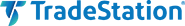### TRADESTATION: APRIL 2021

In his article in this issue, “Detecting High-Volume Breakouts,” author Markos Katsanos introduces an indicator called volume positive negative (VPN) that attempts to minimize entries in false breakouts. The indicator compares volume on “up” days versus the volume on “down” days and is normalized to oscillate between 100 and -100. Shown here is EasyLanguage code for a function, the indicator described in the article, and a sample strategy for educational purposes.

```Function:  _TASC_2021_APR_Fx
// TASC APR 2021
// Detecting High-Volume Breakouts
// Markos Katsanos

inputs:
Period( numericsimple );

variables:
MAV( 0 ),
VP( 0 ),
VN( 0 ),
VPN( 0 );

switch ( BarType )
begin
case 2,3,4: { Daily, Weekly, or Monthly bars }
MAV = Average( Volume, Period );
VP = SummationIf( TypicalPrice -
TypicalPrice > 0.1 *
AvgTrueRange( Period ),
Volume , Period );
VN = SummationIf( TypicalPrice -
TypicalPrice < -0.1 *
AvgTrueRange( Period ),
Ticks , Period );

default: { all other bars }
MAV = Average( Ticks, Period );
VP = SummationIf( TypicalPrice -
TypicalPrice > 0.1 *
AvgTrueRange( Period ),
Ticks , Period );
VN = SummationIf( TypicalPrice -
TypicalPrice < -0.1 *
AvgTrueRange( Period ),
Ticks , Period );
end;

if MAV <= 0 then
MAV = 1;

if Period <> 0 then
VPN = ( VP - VN ) / MAV / Period * 100;

_TASC_2021_APR_Fx = VPN;

Indicator: TASC APR 2021
// TASC APR 2021
// Detecting High-Volume Breakouts
// Markos Katsanos

inputs:
Period( 30 ),
Smooth( 3 ),
VPNCrit( 10 ),
MAB( 30 );

variables:
VPN( 0 ),
MAVPN( 0 );

VPN = XAverage( _TASC_2021_APR_Fx( Period ), Smooth );
MAVPN = Average( VPN, MAB );

if VPN >= VPNCrit then
SetPlotColor( 1, Green )
else
SetPlotColor( 1, Red );

Plot1( VPN, "VPN" );
Plot2( MAVPN, "MA", Green );
Plot3( VPNCrit, "CRIT", Blue );

Strategy:  TASC APR 2021 Strategy
// TASC APR 2021
// Detecting High-Volume Breakouts
// Markos Katsanos

inputs:
Period( 30 ),
Smooth( 3 ),
VPNCrit( 10 ),
MAB( 30 ),
RSILen( 5 ),
MinC( 1 ),
MinVol( 100000 ),
MinVolAvgLen( 5 ),
VolAvgLen( 50 ),
MinVC( 0.5 ),
BarToExitOn( 15 ),
VolDivisor( 1000000 ),
RSIMaxVal( 90 );

variables:
VPN( 0 ),
MAVPN( 0 ),
RSIVal( 0 ),
LQD( false ),

VPN = XAverage( _TASC_2021_APR_Fx( Period ), Smooth );
MAVPN = Average( VPN, MAB );
RSIVal = RSI( Close, RSILen );

switch ( BarType )
begin
case 2,3,4: { Daily, Weekly, or Monthly bars }
// Price, Volume and Liquidity Filter
LQD = Close > MinC
and Average( Volume, MinVolAvgLen ) > MinVol
and Average( Close * Volume, MinVolAvgLen )
/ VolDivisor > MinVC;

BuyCond1 = LQD and
Average( Volume, VolAvgLen ) >
Average( Volume, VolAvgLen );

default: { all other bars }
// Price, Volume and Liquidity Filter
LQD = Close > MinC
and Average( Ticks, MinVolAvgLen ) > MinVol
and Average( Close * Ticks, MinVolAvgLen )
/ VolDivisor > MinVC;

BuyCond1 = LQD and
Average( Ticks, VolAvgLen ) >
Average( Ticks, VolAvgLen );
end;

and VPN crosses above VPNCrit
and RSIVal < RSIMaxVal
and Close > Average( Close, Period ) then
begin
Buy next bar market;
end;

// sell
if VPN crosses under MAVPN
and Close < Highest( Close, 5 )
- 3 * AvgTrueRange( Period ) then
begin
Sell next bar at market;
end;

// time exit
if BarsSinceEntry = BarToExitOn then
Sell ( "Time LX" ) next bar at market;
```

A sample chart is shown in Figure 1.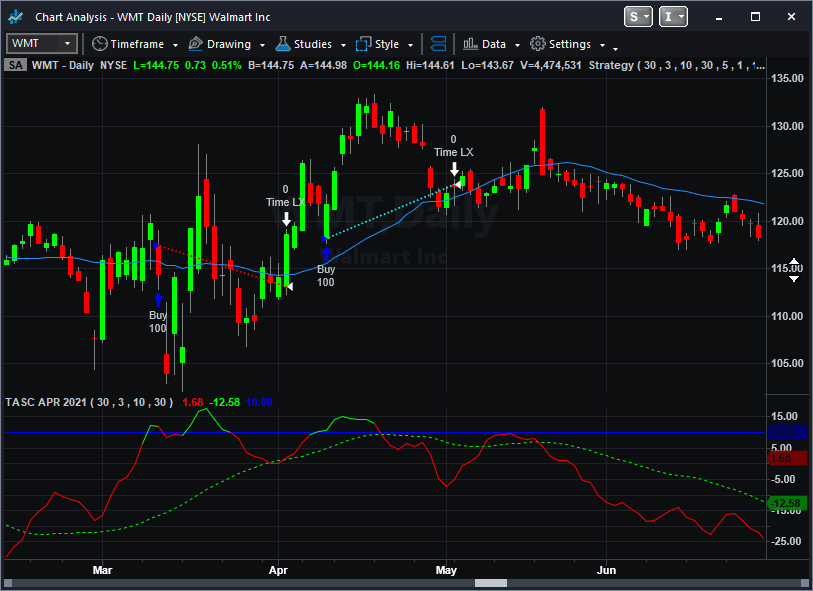FIGURE 1: TRADESTATION. A TradeStation daily chart of Walmart Inc. (WMT) displays the indicator applied in the subgraph and the strategy inserted.

This article is for informational purposes. No type of trading or investment recommendation, advice, or strategy is being made, given, or in any manner provided by TradeStation Securities or its affiliates.

—Chris Imhof

BACK TO LIST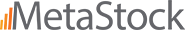### METASTOCK: APRIL 2021

Markos Katsanos’ article in this issue, “Detecting High-Volume Breakouts,” introduces his volume positive negative (VPN) indicator. Here is the formula to add that indicator to MetaStock.

```Volume Positive Negative:

pds:= Input( "Periods", 5, 100, 30);
apr:= Typical();
at:= ATR(pds) * 0.1;
vpd:= If( apr >= Ref(apr, -1) + at, V, 0);
vnd:= If( apr <= Ref(apr, -1) - at, V, 0);
VP:= Sum(vpd, pds);
VN:= Sum(vnd, pds);
VPN:= (((VP - VN)/Mov(V, pds, S))/pds) * 100;
VPN;
Mov(VPN, 3, E)
```

—William Golson
MetaStock Technical Support
www.MetaStock.com

BACK TO LIST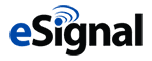### eSIGNAL: APRIL 2021

For this month’s Traders’ Tip, we are providing the “Volume Positive Negative Indicator.efs” study based on the article in this issue by Markos Katsanos, “Detecting High-Volume Breakouts.” The author has designed the indicator to help identify high-volume breakout patterns.

The study contains formula parameters that may be configured through the edit chart window (right-click on the chart and select “edit chart”). A sample chart displaying the indicator is shown in Figure 2.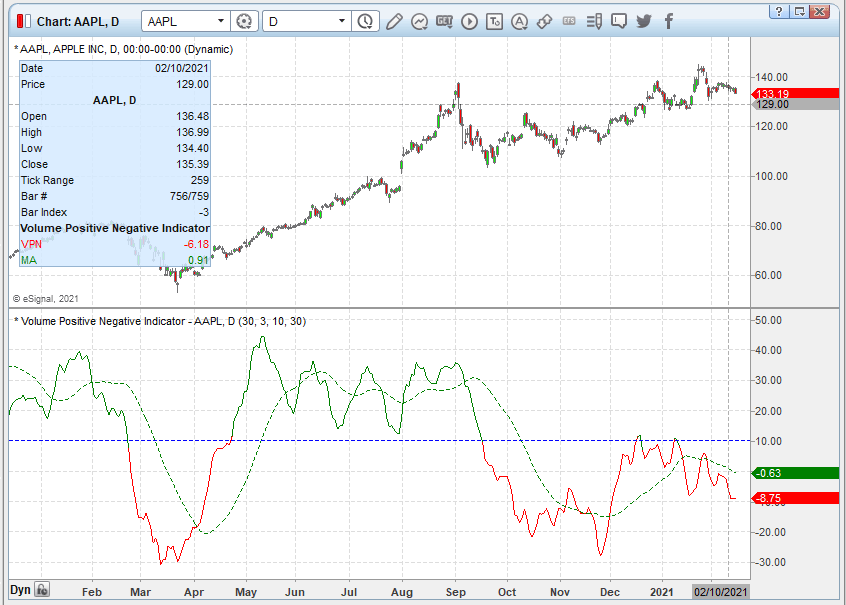FIGURE 2: eSIGNAL. Here is an example of the study plotted on a daily chart of AAPL.

To discuss this study or download a complete copy of the formula code, please visit the EFS library discussion board forum under the forums link from the support menu at www.esignal.com or visit our EFS KnowledgeBase at http://www.esignal.com/support/kb/efs. The eSignal formula script (EFS) is also available for copying & pasting below.

```/**********************************
Provided By:
eSignal is a service mark and/or a registered service mark of Intercontinental Exchange, Inc.
in the United States and/or other countries. This sample eSignal Formula Script (EFS)
is for educational purposes only.
Intercontinental Exchange, Inc. reserves the right to modify and overwrite this EFS file with each new release.

Description:
The Volume Positive Negative (VPN) Indicator
Detecting High-Volume Breakouts
by Markos Katsanos

Version:            1.00  02/11/2021

Formula Parameters:                    Default:
VPPeriod                               30
SMOOTH                                 3
VPNCRIT                                10
MAB                                    30

Notes:
The related article is copyrighted material. If you are not a subscriber
of Stocks & Commodities, please visit www.traders.com.

**********************************/
var fpArray = new Array();
var bInit = false;

function preMain() {
setStudyTitle("Volume Positive Negative Indicator");
setCursorLabelName("VPN", 0);
setCursorLabelName("MA", 1);
setPriceStudy(false);
setDefaultBarFgColor(Color.RGB(0x00,0x94,0xFF), 0);
setDefaultBarFgColor(Color.green, 1);
setPlotType( PLOTTYPE_LINE , 0 );
setPlotType( PLOTTYPE_LINE , 1 );
setDefaultBarStyle(PS_DASH, 1);

var x=0;
fpArray[x] = new FunctionParameter("VPPeriod", FunctionParameter.NUMBER);
with(fpArray[x++]){
setLowerLimit(5);
setUpperLimit(100);
setDefault(30);
}
fpArray[x] = new FunctionParameter("SMOOTH", FunctionParameter.NUMBER);
with(fpArray[x++]){
setLowerLimit(1);
setUpperLimit(10);
setDefault(3);
}
fpArray[x] = new FunctionParameter("VPNCRIT", FunctionParameter.NUMBER);
with(fpArray[x++]){
setLowerLimit(0);
setUpperLimit(40);
setDefault(10);
}
fpArray[x] = new FunctionParameter("MABARS", FunctionParameter.NUMBER);
with(fpArray[x++]){
setLowerLimit(10);
setUpperLimit(200);
setDefault(30);
}

}

var bVersion = null;
var xROCDEV = null;
var xMAROC = null;
var xROC = null;
var xClose = null;

function main(VPPeriod, SMOOTH, VPNCRIT, MABARS) {
if (bVersion == null) bVersion = verify();
if (bVersion == false) return;

if ( bInit == false ) {
xClose = close();
xHigh = high();
xLow = low();
xVolume = volume();
xMA = efsInternal("Calc_MA", xVolume, VPPeriod);
xAVG =  efsInternal("Calc_AVG", xClose, xHigh, xLow);
xMF =  efsInternal("Calc_MF", xAVG);
MC = efsInternal("Calc_MC", VPPeriod);
xVMP = efsInternal("Calc_VMP", xMF, MC, xVolume);
xVP = efsInternal("Calc_VP", xVMP, VPPeriod);
xVMN = efsInternal("Calc_VMN", xMF, MC, xVolume);
xVN = efsInternal("Calc_VP", xVMN, VPPeriod);
xVPN = efsInternal("Calc_VPN", xVP, xVN, xMA, VPPeriod);
VPN = ema(SMOOTH, xVPN);
xMAVPN = sma(MABARS, VPN);
addBand( VPNCRIT, PS_DASH, 1, Color.blue);

bInit = true;
}

if (getCurrentBarCount() <= VPPeriod) return;
if (VPN.getValue(0) >= VPNCRIT) setBarFgColor(Color.green, 0);
else setBarFgColor(Color.red, 0);

return [VPN.getValue(0), xMAVPN.getValue(0)]
}

function Calc_MC(VPPeriod){
var ret = 0;
ret = 0.1 * atr(VPPeriod);
return ret;
}

function Calc_VPN(xVP, xVN, xMA, VPPeriod){
var ret = 0;
ret = (xVP.getValue(0) - xVN.getValue(0)) / xMA.getValue(0) / VPPeriod * 100;
return ret;
}

function Calc_VMN(xMF, MC, xVolume){
var ret = 0;
if (xMF.getValue(0) < -MC.getValue(0)) return xVolume.getValue(0);
return 0;
}

function Calc_VP(xVMP, VPPeriod){
var ret = 0;
for (var i = 0; i < VPPeriod; i++){
ret = ret + xVMP.getValue(-i);
}
return ret;
}

function Calc_VMP(xMF, MC, xVolume){
var ret = 0;
if (xMF.getValue(0) > MC.getValue(0)) return xVolume.getValue(0);
return 0;
}

function Calc_MF(xAVG){
var ret = 0;
ret = xAVG.getValue(0) - xAVG.getValue(-1);
return ret;
}

function Calc_MA(xVolume, VPPeriod){
var Sum = 0;
for (var i = 0; i < VPPeriod; i++){
if (xVolume.getValue(-i) == null || xVolume.getValue(-i) == 0) {
Sum = Sum + 1
}
else Sum = Sum + xVolume.getValue(-i)
}
var ret = Sum / VPPeriod;
if (ret > 0) return ret;
return 1;
}

function Calc_AVG(xClose, xHigh, xLow){
var ret = 0;
ret = (xClose.getValue(0) + xHigh.getValue(0) + xLow.getValue(0)) / 3;
return ret;
}

function verify(){
var b = false;
if (getBuildNumber() < 779){

drawTextAbsolute(5, 35, "This study requires version 10.6 or later.",
Color.white, Color.blue, Text.RELATIVETOBOTTOM|Text.RELATIVETOLEFT|Text.BOLD|Text.LEFT,
null, 13, "error");
Color.white, Color.blue, Text.RELATIVETOBOTTOM|Text.RELATIVETOLEFT|Text.BOLD|Text.LEFT,
return b;
}
else
b = true;

return b;
}
```

—Eric Lippert
eSignal, an Interactive Data company
800 779-6555, www.eSignal.com

BACK TO LIST### THINKORSWIM: APRIL 2021

We have put together a study based on the article by Markos Katsanos in this issue titled “Detecting High-Volume Breakouts,” which introduces the volume positive negative (VPN) indicator. We built the study referenced by using our proprietary scripting language, thinkscript. To ease the loading process, simply click on http://tos.mx/eFC5Tjn or enter it into the address into setup→open shared item from within thinkorswim then choose view thinkScript study and name it “VPN_indicator” or whatever you like and can identify. You can then add the study to your charts from the edit studies menu from within the charts tab.

The example chart in Figure 3 shows the study on a chart of IDEX over a period from June 2019 till November 2020. Please see Markos Katsanos’ article in this issue for more details on how to utilize the indicator.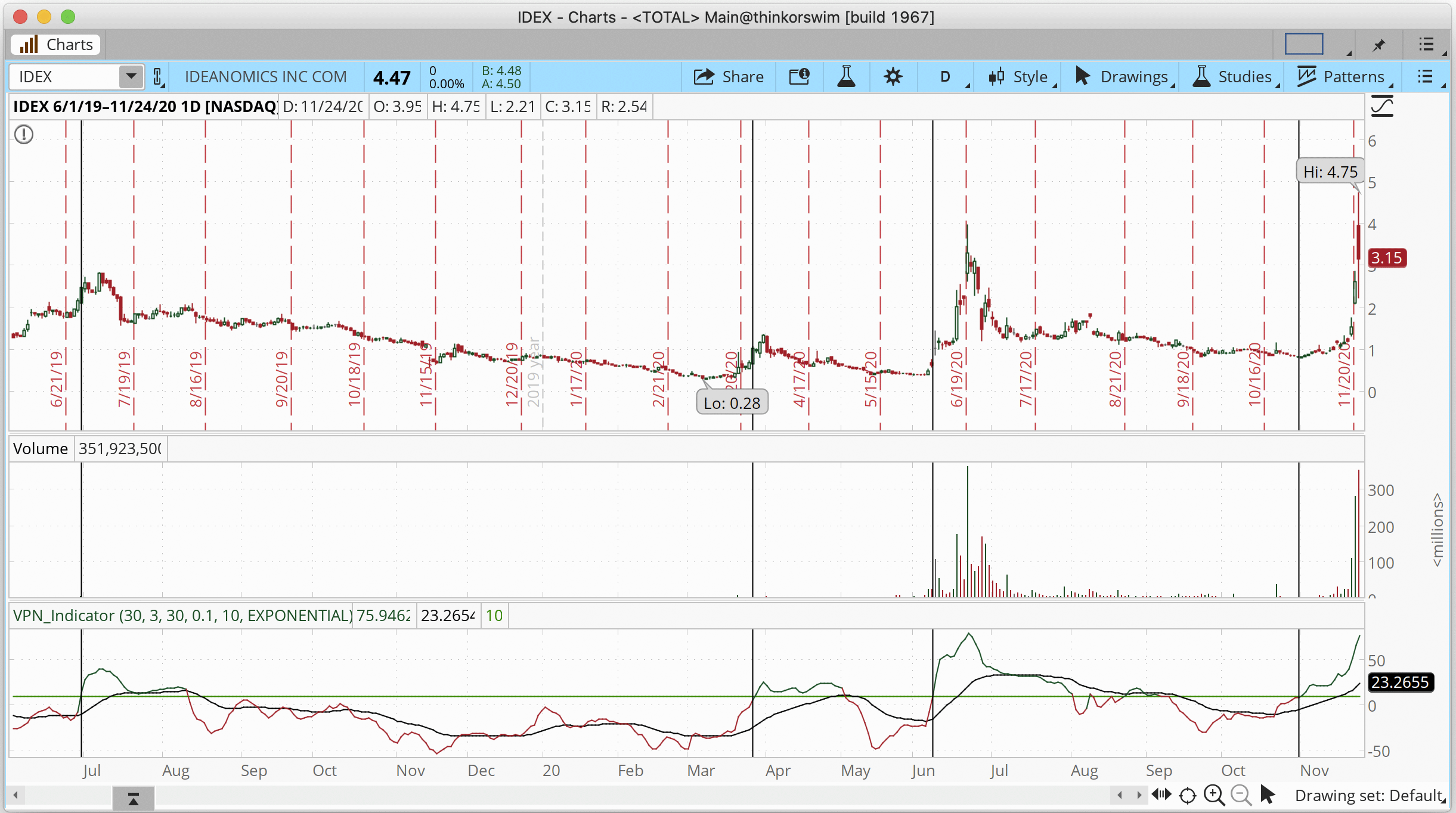FIGURE 3: THINKORSWIM. The study is shown plotted on a chart of IDEX from June 2019 till November 2020.

—thinkorswim
A division of TD Ameritrade, Inc.
www.thinkorswim.com

BACK TO LIST### WEALTH-LAB.COM: APRIL 2021

We added the VPN oscillator based on Markos Katsanos’s article in this issue to the TASCIndicators library (built-in Wealth-Lab 7). To execute the trading system, paste the code below into a new C# strategy window.

The code is available for copying and pasting here:

```using WealthLab.Backtest;
using System;
using WealthLab.Core;
using WealthLab.Indicators;
using WealthLab.TASC;
using System.Drawing;
using System.Collections.Generic;

namespace WealthScript1
{
public class VPNStrategy : UserStrategyBase
{
public VPNStrategy()
{
AddParameter("VPN Period", ParameterTypes.Int32, 30, 5, 100, 10);
AddParameter("Smoothing Period", ParameterTypes.Int32, 3, 1, 10, 1);
}

public override void Initialize( BarHistory bars)
{
period = Parameters.FindName("VPN Period").AsInt;
smoothPeriod = Parameters.FindName("Smoothing Period").AsInt;
vpn = VPN.Series(bars, period, smoothPeriod);
maVPN = SMA.Series(vpn, period);
maVolume = SMA.Series(bars.Volume, 50);
rsi = RSI.Series(bars.Close, 5);
maPrice = SMA.Series(bars.Close, period);
atr = ATR.Series(bars, 14);

PlotTimeSeries( vpn, "VPN", "VPN", Color.Black);
PlotIndicatorLine( maVPN as IndicatorBase, Color.Blue, 1);
DrawHorzLine( 80, Color.Green, 1, default, "VPN");
DrawHorzLine(-80, Color.Red, 1, default, "VPN");

for (int i = 0; i < bars.Count; i++)
{
SetSeriesBarColor( vpn, i, vpn[i] > 0 ? Color.Green : Color.Red);
}

index = GetHistory( bars, "IWM");
indexRoc = ROC.Series(index.Close, 10);
indexMA = SMA.Series(index.Close, 150);

PlotBarHistory( index, "IWM");
PlotTimeSeries( vpn, "VPN", "VPN", Color.Black);
PlotTimeSeries( indexMA, "Index MA", "IWM", Color.Black);

StartIndex = 150;
}

public override void Execute(BarHistory bars, int bar)
{
if (!HasOpenPosition(bars, PositionType.Long))
{
if(vpn.CrossesOver(10,bar))
if(maVolume[bar] > maVolume[bar-50])
if(rsi[bar] < 90)
if(bars.Close[bar] > maPrice[bar])
}
else
{
if(bar > LastPosition.EntryBar + 15)
ClosePosition( LastPosition, OrderType.Market, 0, "Time-based");
else
{
if(vpn.CrossesUnder(SMA.Series(vpn,smoothPeriod), bar) && bars.Close[bar] < Highest.Value( bar,bars.High,5)+atr[bar]*3.0)							if(indexRoc[bar] < -15 && index[bar] < indexMA[bar])
ClosePosition( LastPosition, OrderType.Market, 0, "Normal exit");
}
}
}

TimeSeries vpn, maVPN, maPrice, maVolume, rsi, atr, indexRoc, indexMA;
int period, smoothPeriod;
BarHistory index;
}
}
```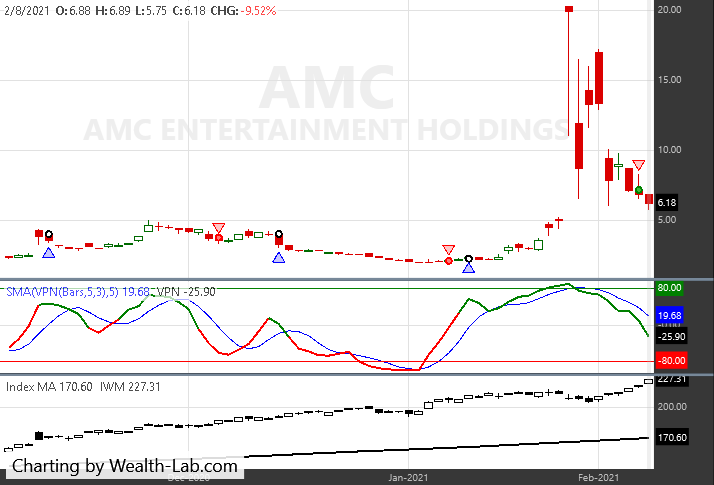FIGURE 4: WEALTH-LAB. Sample trade signals are shown on a chart of AMC Entertainment (AMC) based on the VPN indicator.

—Gene Geren (Eugene)
Wealth-Lab team
www.wealth-lab.com

BACK TO LIST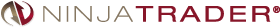### NINJATRADER: APRIL 2021

The VPN indicator and VPNSystem strategy based on Markos Katsanos’ article in this issue are available for download at the following links for NinjaTrader 8 and NinjaTrader 7:

Once the file is downloaded, you can import the indicator/strategy into NinjaTader 8 from within the control center by selecting Tools → Import → NinjaScript Add-On and then selecting the downloaded file for NinjaTrader 8. To import into NinjaTrader 7, from within the control center window, select the menu File → Utilities → Import NinjaScript and select the downloaded file.

You can review the indicator and strategy source code in NinjaTrader 8 by selecting the menu New → NinjaScript Editor → Indicators or Strategies folder from within the control center window and selecting the VPN or VPNSystem file. You can review the indicator’s source code in NinjaTrader 7 by selecting the menu Tools → Edit NinjaScript → Indicator or Strategy from within the control center window and selecting the VPN or VPNSystem file.

A NinjaTrader chart giving an example of the indicator and strategy are shown in Figure 5.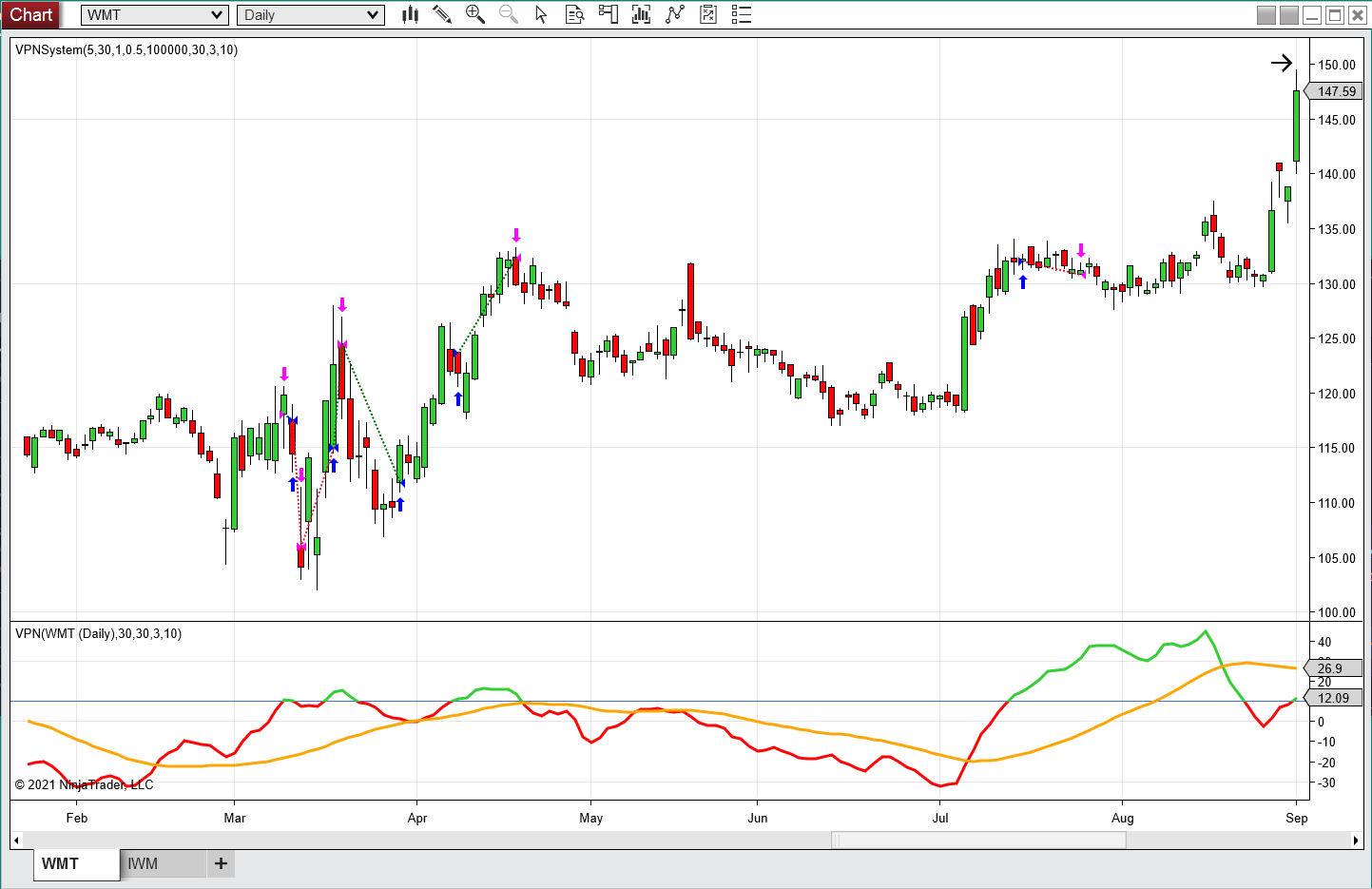FIGURE 5: NINJATRADER. The VPN indicator and strategy are displayed on a daily WMT chart from February 2020 to September 2020.

NinjaScript uses compiled DLLs that run native, not interpreted, to provide you with the highest performance possible.

—Chris Lauber

BACK TO LIST### NEUROSHELL TRADER: APRIL 2021

The volume positive negative (VPN) indicator described by Markos Katsanos in his article in this issue can be easily implemented with a few of NeuroShell Trader’s 800+ indicators. Simply select new indicator from the insert menu and use the indicator wizard to create the following indicators:

```MF	Momentum( Avg3( High, Low, Close ), 1)
MC	Mul2( 0.1, ATR( High, Low, Close, 30 ) )
VP	Sum( IfThenElse( A>B( MF,  MC ), Volume, 0 ), 30 )
VN	Sum( IfThenElse( A<B( MF, Neg(MC) ), Volume, 0), 30 )
VPN	ExpAvg( Mul2( Divide( Divide( Sub( VP, VN ), Avg( Volume, 30 ) ), 30), 100 ), 3 )
MAVPN	Avg( VPN, 30 )
```

To implement the volume positive negative trading system, select new trading strategy from the insert menu and enter the following in the appropriate locations of the trading strategy wizard:

```BUY LONG CONDITIONS: [All of which must be true]
CrossAbove(VPN(High,Low,Close,Volume,30,3),10)
A>B(Momentum(Avg(Volume,50),50),0)
A<B(RSI(Close,5),90)
A>B(Close,Avg(Close,30))

SELL LONG CONDITIONS: [1 of which must be true]
And2(CrossBelow(VPN(High,Low,Close,Volume,30,3), Avg(VPN(High,Low,Close,Volume,30,3),30)),
A<B(Close, Sub(Max(High,5),Mul2(3,ATR(High,Low,Close,30)))))
And2(A<B(Russell 2000 Close, Avg(Russell 2000 Close,50)),
A<B(%Change(Russell 2000 Close,10),-15))
```

NeuroShell Trader users can go to the Stocks & Commodities section of the NeuroShell Trader free technical support website to download a copy of this or any previous Traders’ Tips.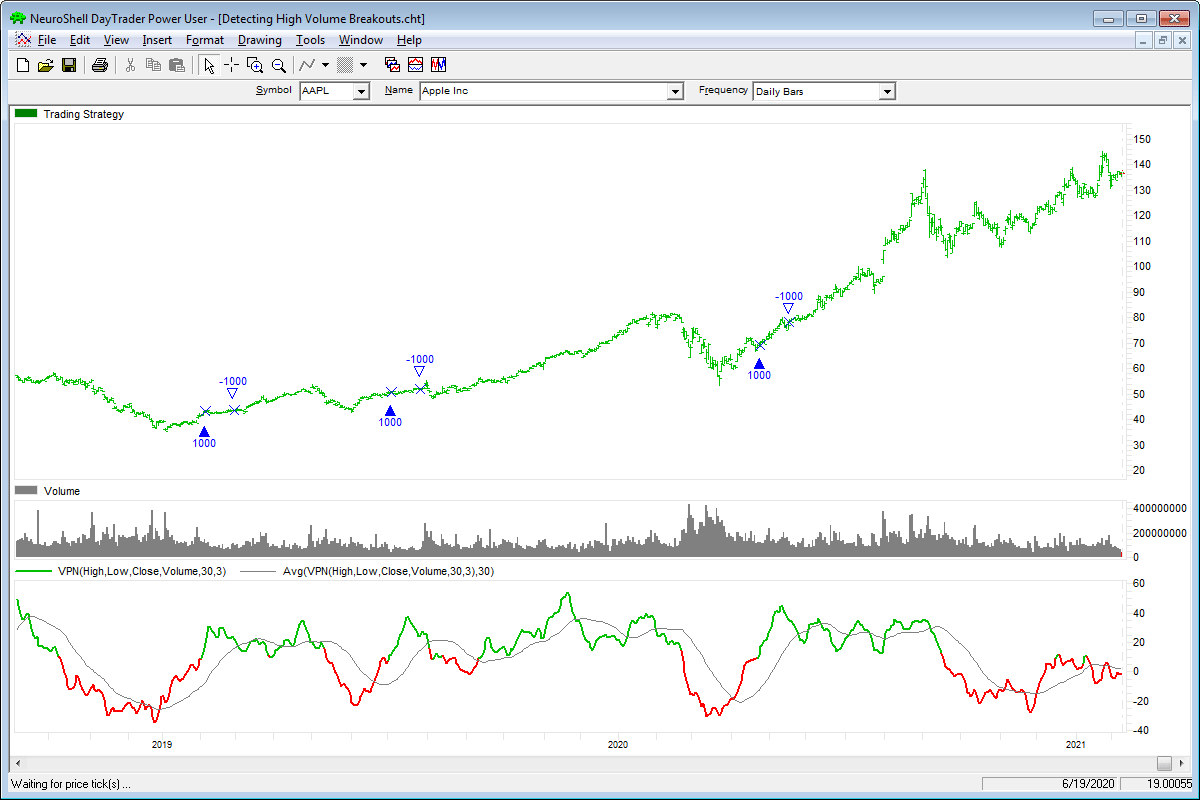FIGURE 6: NEUROSHELL TRADER. This NeuroShell Trader chart displays the volume positive negative indicator and trading system.

—Marge Sherald, Ward Systems Group, Inc.
301 662-7950, sales@wardsystems.com
www.neuroshell.com

BACK TO LIST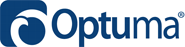### OPTUMA: APRIL 2021

Here’s a formula for implementing in Optuma the volume positive negative (VPN) indicator as described in Markos Katsanos’ article in this issue, “Detecting High-Volume Breakouts.” The formula is for VPN(30):

```\$PERIOD=30;
P1 = MA(BARS=1, CALC=HLC);
MC = ATR(MULT=0.10, BARS=1);
MAV = MA(VOLUME(), BARS=\$PERIOD);
//Positive Volume;
VMP = IF(P1 > P1+MC, VOLUME(),0);
VP= ACC(VMP, RANGE=Look Back Period, BARS=\$PERIOD);
//Negative Volume;
VMN = IF(P1 < P1-MC, VOLUME(),0);
VN= ACC(VMN, RANGE=Look Back Period, BARS=\$PERIOD);
//Calc Smooth VPN;
VPN = (VP-VN)/MAV/30*100;
MA(VPN, BARS=3, STYLE=Exponential, CALC=Close)
```

The bar/volume colors for positive, negative, and neutral (black) volume are based on the rules given in the article. Blue vertical lines are when VPN crosses above 10. A sample chart is shown in Figure 7.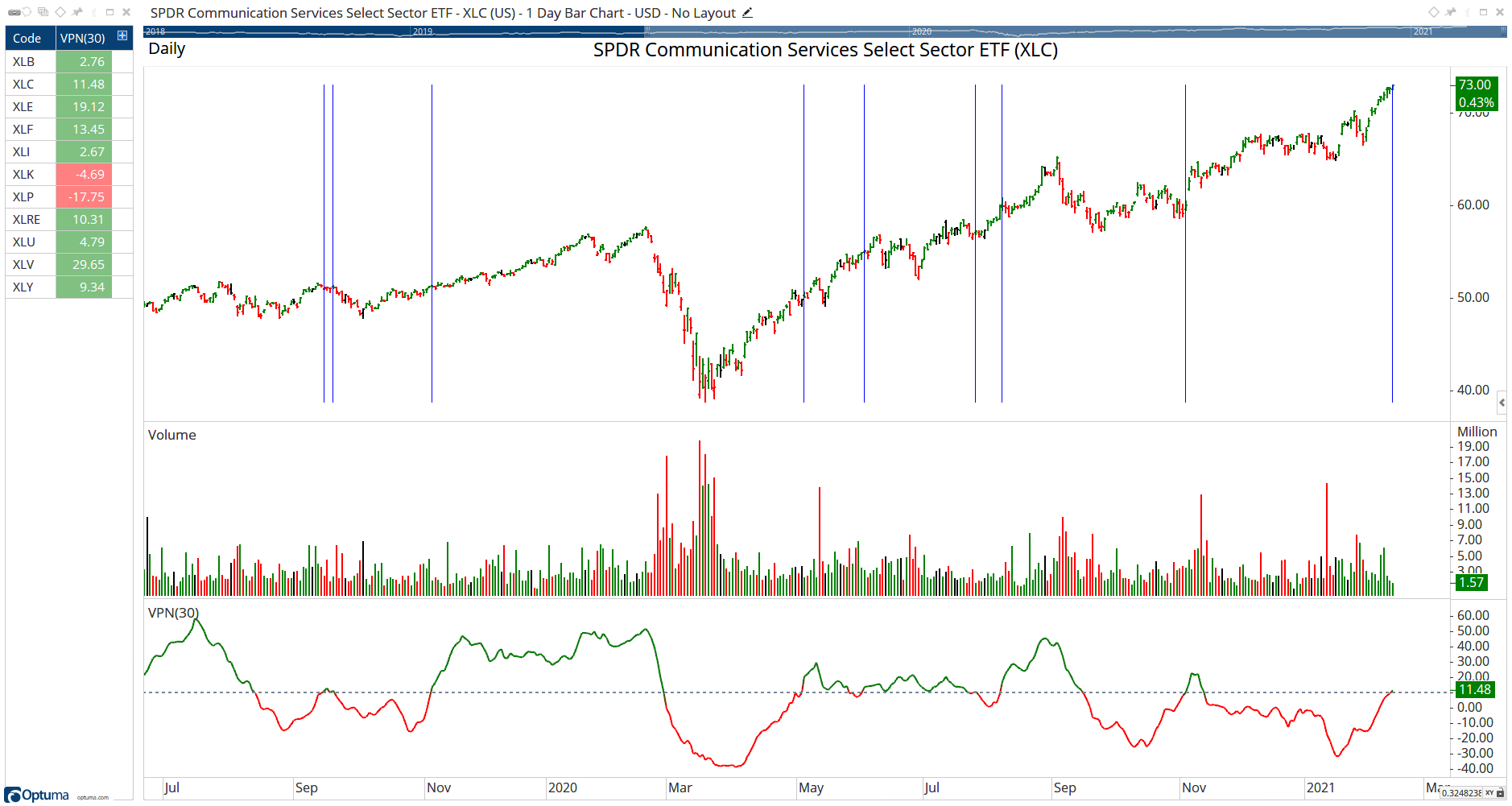FIGURE 7: OPTUMA. This sample chart displays the volume positive negative (VPN) indicator on XLC (SPDR Communications Services Select Sector ETF).

BACK TO LISTThe importable TradersStudio file based on Markos Katsanos’s article in this issue, “Detecting High-Volume Breakouts,” can be obtained on request via email to info@TradersEdgeSystems.com. The code is also available here:

```'Detecting High-Volume Breakouts
'Author: Markos Katsanos, TASC April 2021
'Coded by: Richard Denning, 02/18/2021

function VPN(period,smoLen)
'INPUTS:
'period = 30
'smoLen = 3
'vpnCrit = 10
'maLen = 30
Dim Price As BarArray
Dim MAVol As BarArray
Dim MAV As BarArray
Dim MF As BarArray
Dim TR As BarArray
Dim ATR As BarArray
Dim MC As BarArray
Dim VMP As BarArray
Dim VP As BarArray
Dim VMN As BarArray
Dim VN As BarArray

'FORMULAS:
MAVol = Average(V,period)
MAV = IFF(MAVol>0,MAVol,1)
Price = ( High + Low + Close )/3
MF = Price - Price
TR = max(H-L,max(C-L,H-C))
ATR = Average(TR,period)
MC = 0.1*ATR
VMP = IFF(MF > MC, V, 0)
VP = summation(VMP,period)
VMN = IFF(MF < -MC, V, 0)
VN = summation(VMN,period)
VPN = (XAverage(((VP-VN)/MAV/period),smoLen))*100
'------------------------------------------------
Sub VPN_IND(period,smoLen,vpnCrit,maLen)
'INPUT DEFAULTS:
'period = 30
'smoLen = 3
'vpnCrit = 10
'maLen = 30
Dim theVPN As BarArray
Dim maVPN As BarArray
theVPN = VPN(period,smoLen)
maVPN = Average(theVPN,maLen)
plot1(theVPN)
plot2(maVPN)
plot3(vpnCrit)
End Sub
'------------------------------------------------
```

Code for the VPN indicator is provided in the function “VPN” along with the plot indicator “VPN_IND.” Figure 8 shows the indicator on a chart of Apple Inc. (AAPL).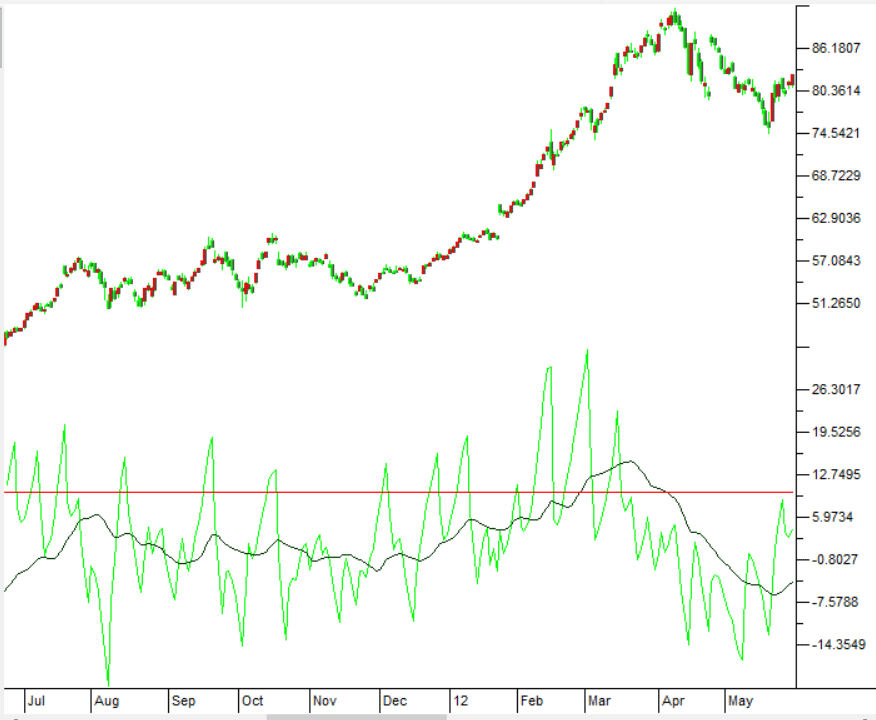FIGURE 8: TRADERSSTUDIO. The VPN indicator is shown on a chart of Apple Inc. (AAPL).

—Richard Denning

BACK TO LIST### AIQ: APRIL 2021

The importable AIQ EDS file based on Markos Katsanos’ article in this issue, “Detecting High-Volume Breakouts,” can be obtained on request via email to info@TradersEdgeSystems.com. The code is also available here:

```!Detecting High-Volume Breakouts
!Author: Markos Katsanos, TASC April 2021
!Coded by: Richard Denning, 02/18/2021

!INPUTS:
period is 30.
smoLen is 3.
vpnCrit is 10.
maLen is 30.
V is [volume].

!FORMULAS:
MAVol is simpleavg(V,period).
MAV is iff(MAVol>0,MAVol,1).
Avg is ([High]+[Low]+[Close])/3.
MF is Avg - valresult(Avg,1).
ATR is simpleavg(max( [high]-[low],
max(val([close],1)-[low],
[high]-val([close],1))),period).
MC is 0.1*ATR.
VMP is iff(MF > MC, V, 0).
VP is sum(VMP,period).
VMN is iff(MF < -MC, V, 0).
VN is sum(VMN,period).
VPN is (expavg(((VP - VN) / MAV / period),smoLen))*100.
MAVPN is simpleavg(VPN,maLen).
```

Code for the VPN indicator is set up in the AIQ code file. Figure 9 shows the indicator on a chart of Tesla Motors Inc (TSLA).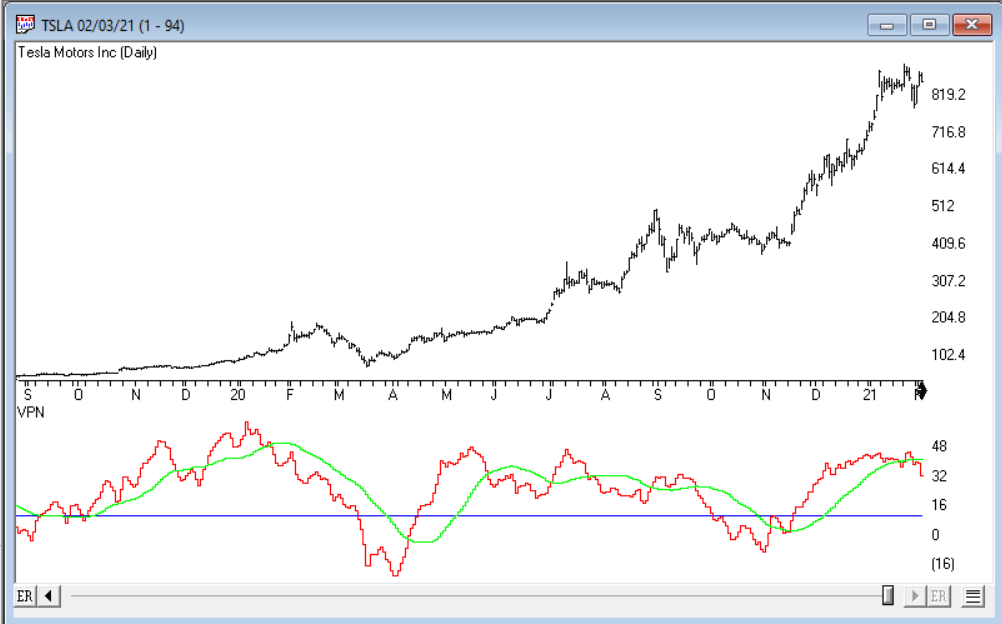FIGURE 9: AIQ. The VPN indicator is shown on a chart of Tesla Motors Inc. (TSLA).

—Richard Denning
for AIQ Systems

BACK TO LIST### THE ZORRO PROJECT: APRIL 2021

It is estimated that about 6,000 different technical indicators have been developed over the years. However, few of them are based on volume. In this issue, Markos Katsanos proposes a new indicator for detecting high-volume breakouts, and he tests it with a trading system based on the Russell 2000 index.

The volume positive negative (VPN) indicator calculates the difference of up day volume and down day volume, divided by the total volume. A day is an up day when its typical price—the average of high, low, and close—is higher than yesterday’s typical price plus 1/10 ATR. The opposite is true for down days. The result is multiplied by 100 and smoothed with a 3-day EMA. The C code for this indicator is:

```var VPN(vars Volumes,int Period)
{
var Dist = 0.1*ATR(Period);
vars TypPrices = series(TypPrice());
var Vp=0,Vn=0,Vtotal=0; int i;
for(i=0; i<Period; i++) {
if(TypPrices[i] > TypPrices[i+1] + Dist)
Vp += Volumes[i];
else if(TypPrices[i] < TypPrices[i+1] - Dist)
Vn += Volumes[i];
Vtotal += Volumes[i];
}
return EMA(100*(Vp-Vn)/Vtotal,3);
}
```

In his article, the author shows a chart with the VPN applied to Ideanomics Inc. (IDEX), a supplier to electric vehicles. Here is the code for replicating his chart:

```void run()
{
BarPeriod = 1440;
StartDate = 20190501;
EndDate = 20201231;

asset("IDEX");
vars Volumes = series(marketVol());
plot("TPR",VPN(Volumes,30),NEW,RED);
plot("Threshold",10,0,BLACK);
}
```

The resulting chart is displayed with a threshold line at 10% (Figure 10). The VPN crossing this line is designed to be a buy signal. We can see that the signals are valid, but a bit late. They catch only the top of the price peaks. It’s possible that it would be better to smooth the indicator not with an EMA but with a filter with less lag, such as a two-pole lowpass filter or one of John Ehlers’ zero-lag filters.FIGURE 10: ZORRO PROJECT. Here, the VPN indicator is applied to a chart of Ideanomics Inc. (IDEX), a supplier to electric vehicles.

In our replication of his test we’ll use walk-forward optimization. As in the article, we invest 10,000 per stock and allow a maximum of 10 open positions. For comparison with a benchmark, we invest the same amount in a Russell 2000 ETF (IWM). The C code for the trading system is as follows:

```void run()
{
setf(TrainMode,SETFACTORS); // skip OptimalF calculation
setf(PlotMode,PL_ALL|PL_BENCHMARK);
set(PARAMETERS);
NumWFOCycles = 5;
NumCores = -2;
BarPeriod = 1440;
StartDate = 2011;
EndDate = 2021;
LookBack = 150;
UpdateDays = 5; // don't download all again every day

assetList("AssetsRussell2000");
assetAdd("IWM","STOOQ:IWM.US"); // Russell2000 index ETF

int Period = optimize(30,30,40,10);
var Threshold = optimize(10,10,20,10);

// Sell after 15 days,
// or when a Russell2000 15% drop and a value below the 150-day SMA indicates meltdown.
asset("IWM");
int Meltdown = ROC(seriesC(),10) < -15 && priceClose() < SMA(seriesC(),150);

// Use Russell2000 index (IWM) as benchmark
static var StartPrice;
if(is(LOOKBACK)) StartPrice = priceClose();
var Benchmark = 10*10000/priceClose() * (priceClose()-StartPrice);
plot("Russell2000",Benchmark,AXIS2,PURPLE);

for(listed_assets)
{
asset(Asset);
if(Asset == "IWM") continue; // don't trsde the index
vars Volumes = series(marketVol(0),100);
vars Prices = series(priceClose(),30);
vars Signals = series(VPN(Volumes,Period),2);

// Filter out when price was below 1
// or 5-day average volume below 100000
// or the 5-day average product of price and volume below 500000.
bool Filter = Prices < 1
|| SMA(Volumes,5) < 100000
|| SMA(series(Volumes*Prices,5),5) < 500000;

// buy when the VPN crosses over 10%
// and its 50-day volume average is above its previous 50-day average,
// its 5-day RSI is below 90, and the price is above its 30-day SMA.
bool Buy = crossOver(Signals,Threshold)
&& SMA(Volumes,50) > SMA(Volumes+50,50)
&& RSI(Prices,5) < 90
&& Prices > SMA(Prices,30);

// Sell when the VPN crosses below 30-day SMA while price is more than 3 ATR below 5-day high,
// or when a market meltdown is immiment
bool Sell = (crossUnder(Signals,SMA(Signals,30))
&& Prices < HH(5) - 3*ATR(5)) || Meltdown;

if(!Filter && Buy && !Sell && (Train || NumLongTotal < 10))
enterLong(10000/Prices);
else if(Sell)
exitLong();
}
}
```

We have added code comments for the various buy, sell, and filter conditions. The first start of this system will be slow because the histories of all 2000 stocks are downloaded. The Russell 2000 asset list is set up to load them from Yahoo. Subsequent starts are faster. The profit curves are plotted in the chart in Figure 11, blue for the trading system and purple for the benchmark.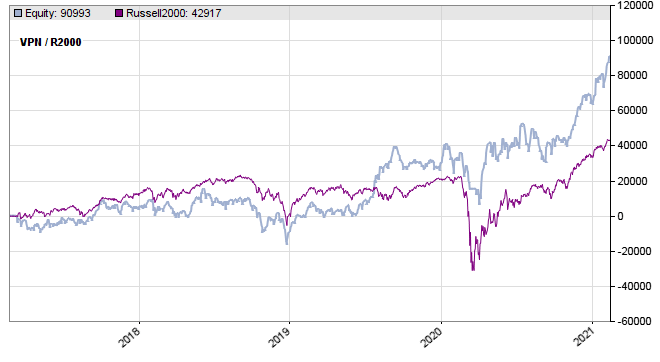FIGURE 11: ZORRO PROJECT. Here is a sample equity curve for the system against a benchmark.

We used 10 years of history, but the backtest started only in 2017 due to the preceding WFO training periods.

The VPN indicator and the trading system can be downloaded from the 2021 script repository on https://financial-hacker.com. The Zorro platform can be downloaded from https://zorro-project.com.

—Petra Volkova
The Zorro Project by oP group Germany
https://zorro-project.com

BACK TO LIST

### MICROSOFT EXCEL: APRIL 2021

In his article in this issue, “Detecting High-Volume Breakouts,” Markos Katsanos presents an indicator that looks at the balance of up and down volume as the first step in identifying a breakout trading opportunity.

Figures 12 and 13 show a sample chart of the indicator based on the formula.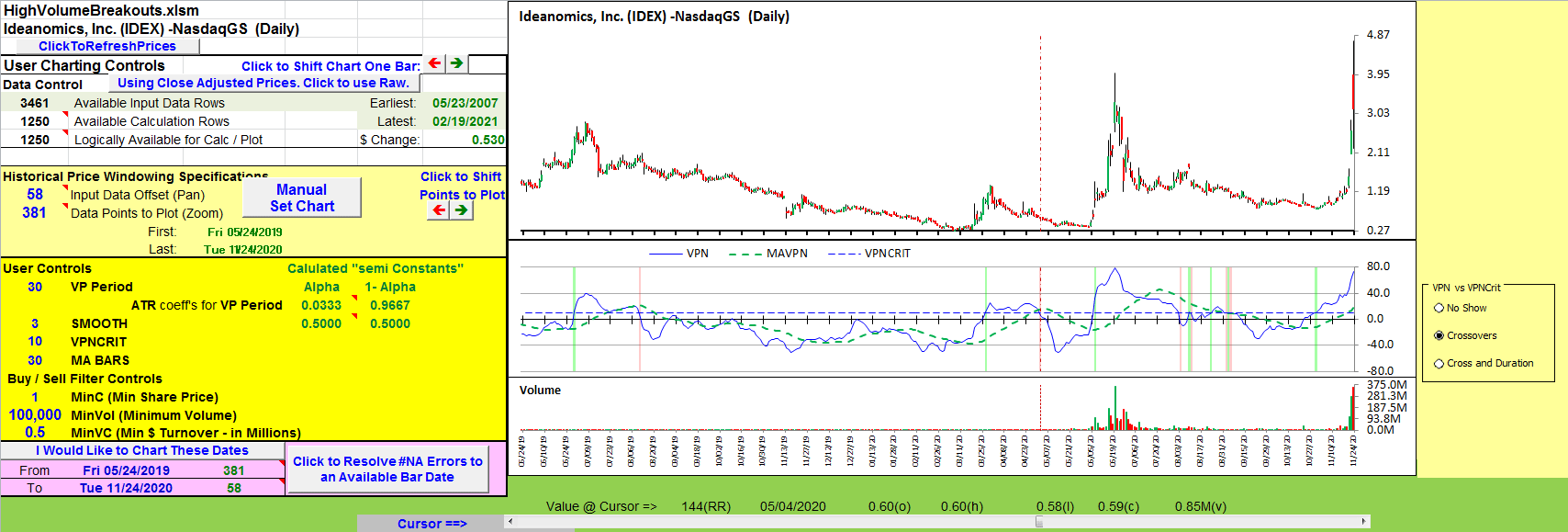FIGURE 12: EXCEL. Green bars mark where the VPN crosses above the user-specified VPNCrit threshold marking possible breakouts.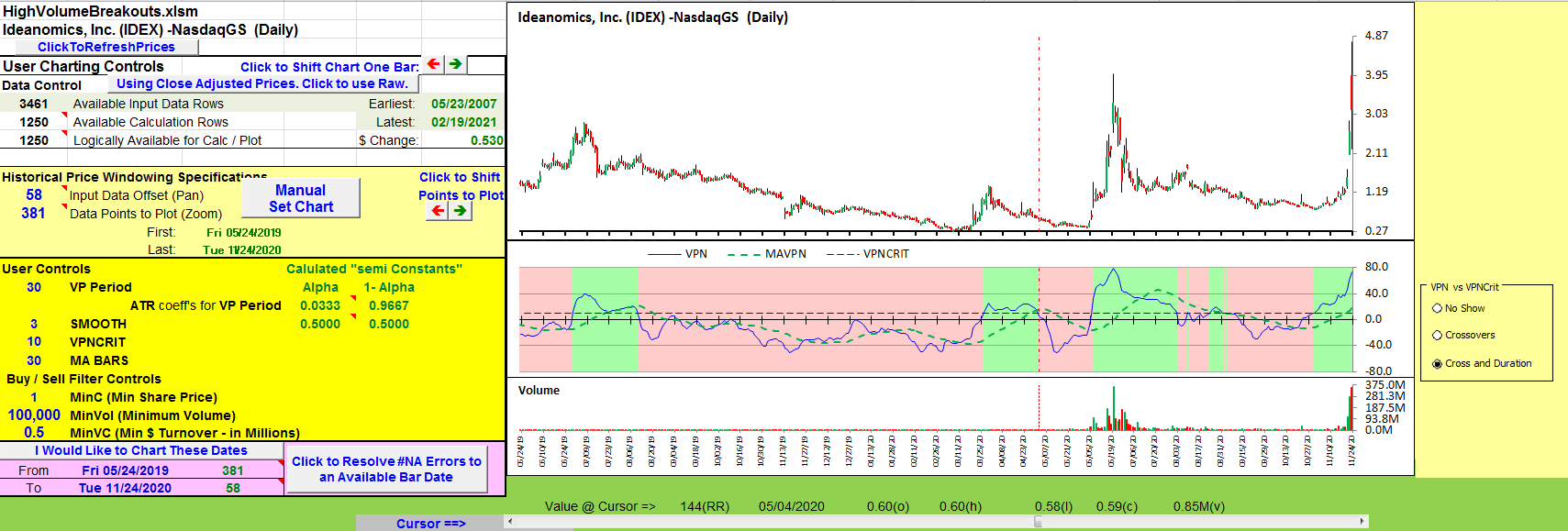FIGURE 13: EXCEL. Here, the chart uses full-on shading while the VPN is above or below the VPNCrit value.

A spreadsheet implementing the formula given in the article can be found here. To download the spreadsheet, please follow these steps:

• Right-click on the Excel file link, then
• Select “save as” (or “save target as”) to place a copy of the spreadsheet file on your hard drive.

—Ron McAllister
Excel and VBA programmer
rpmac_xltt@sprynet.com

BACK TO LIST

Originally published in the April 2021 issue of
Technical Analysis of STOCKS & COMMODITIES magazine.